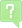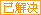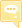问题# c++语言程序最佳回答
#include iostreamusing namespace std;int main(){long long n,m,nm,t,r;cinnm;nm=n*m;if(mn){ t=m; m=n; n=t;}r=m%n;while (r!=0){ m=n; n=r; r=m%n;}cout"最大公约数为"nendl;cout"最小公倍数为"nmnendl;return 0;}